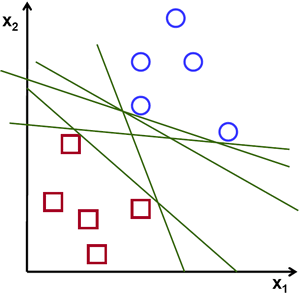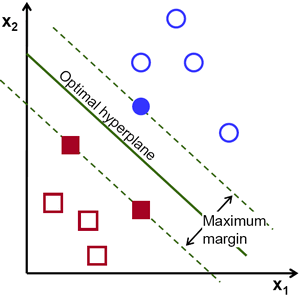# www.HadoopExam.com

HadoopExam Learning Resources

## Support Vector Machine (SVM)

Support Vector Machine:  In machine learning, support vector machines (SVMs, also support vector networks) are supervised learning models with associated learning algorithms that analyze data and recognize patterns, used for classification and regression analysis. Given a set of training examples, each marked as belonging to one of two categories, an SVM training algorithm builds a model that assigns new examples into one category or the other, making it a non-probabilisticbinarylinear classifier. An SVM model is a representation of the examples as points in space, mapped so that the examples of the separate categories are divided by a clear gap that is as wide as possible. New examples are then mapped into that same space and predicted to belong to a category based on which side of the gap they fall on. In addition to performing linear classification, SVMs can efficiently perform a non-linear classification using what is called the kernel trick, implicitly mapping their inputs into high-dimensional feature spaces.

A support vector machine constructs a hyperplane or set of hyperplanes in a high- or infinite- imensional space, which can be used for classification, regression, or other tasks. Intuitively, a good separation is achieved by the hyperplane that has the largest distance to the nearest training data point of any class (so-called functional margin), since in general the larger the margin the lower the generalization error of the classifier.

SVM is used for: classification, regression, and anomaly detection

More detail on SVM :

A Support Vector Machine (SVM) is a discriminative classifier formally defined by a separating hyperplane. In other words, given labeled training data (supervised learning), the algorithm outputs an optimal hyperplane which categorizes new examples. In which sense is the hyperplane obtained optimal? Let’s consider the following simple problem: For a linearly separable set of 2D-points which belong to one of two classes, find a separating straight line.Note : In this example we deal with lines and points in the Cartesian plane instead of hyperplanes and vectors in a high dimensional space. This is a simplification of the problem.It is important to understand that this is done only because our intuition is better built from examples that are easy to imagine. However, the same concepts apply to tasks where the examples to classify lie in a space whose dimension is higher than two. In the above picture you can see that there exists multiple lines that offer a solution to the problem. Is any of them better than the others? We can intuitively define a criterion to estimate the worth of the lines:

A line is bad if it passes too close to the points because it will be noise sensitive and it will not generalize correctly. Therefore, our goal should be to find the line passing as far as possible from all points.

Then, the operation of the SVM algorithm is based on finding the hyperplane that gives the largest minimum distance to the training examples. Twice, this distance receives the important name of margin within SVM’s theory. Therefore, the optimal separating hyperplane maximizes the margin of the training data.SVM can model complex, real-world problems such as text and image classification, hand-writing recognition, and bioinformatics and biosequence analysis. SVM performs well on data sets that have many attributes, even if there are very few cases on which to train the model.

Visit Home Page : http://hadoopexam.com for more detail . As you are not blacklisted user.